﻿ 数据洞察：能源概况的历史演变和预测

# 数据洞察：能源概况的历史演变和预测Data Insights: Historical Evolution and Forecast of Energy Profile

Abstract: Energy production and use is a major part of any economy. California (CA), Arizona (AZ), New Mexico (NM), and Texas (TX) hope to form a realistic new energy compact that focuses on improving the use of clean and renewable energy. This article believes that determining the energy profile is to select and summarize important data, and at the same time realize the visualization of the data, and qualitatively draw the energy profiles of the states. In this paper, a longitudinal analysis of it is made to find out its historical evolution rules and predict future values.

1. 问题的引出

http://www.comap.com/undergraduate/contests/mcm/contests/2018/problems/2018_MCM-ICM_Problems.zip。

2. 符号说明

3. 原始数据检验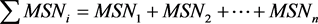,

$\text{error}=\frac{\sum MS{N}_{i}-\left(MS{N}_{1}+MS{N}_{2}+\cdots +MS{N}_{n}\right)}{\sum MSN}$ ,Table 2. Raw data test results

4. 模型的建立与求解

4.1. 各州能量概况

4.1.1. 重要数据的选择与汇总

Ø 能源的消耗量

$TETCBZZ=FFTCBZZ+NUETBZZ+RETCBZZ+ELNIBZZ+ELISBZZ$

$FFTCB=CLTCB+NNTCB+PMTCB$ ,

1) ELNIB：对比于TETCB中的其他元素，ELNIB的数据值占比过小，均小于1%；

2) ELISB：出现负值，且数值较大；

3) 将ELNIB与ELISB从TETCB中剔除，产生新的TETCB，即

$\begin{array}{c}TETCB\left(new\right)=TETCB\left(original\right)-ELNIB-ELISB\\ =FFTCB+NUETB+RETCB\\ =CLTCB+NNTCB+PMTCB+NUETB+RETCB\end{array}$

(注：下文所指的TETCB皆指TETCB(new))。

$Dat{a}_{1}\left(percentage\right)=\frac{Dat{a}_{1}\left(value\right)}{\underset{i=1}{\overset{n}{\sum }}Dat{a}_{i}\left(value\right)}$ ,

$\begin{array}{l}CLTCB\left(percentage\right)\\ =\frac{CLTCB\left(value\right)}{CLTCB\left(value\right)+NNTCB\left(value\right)+PMTCB\left(value\right)+NUETB\left(value\right)+RETCB\left(value\right)}\\ =\frac{CLTCB\left(value\right)}{TETCB\left(value\right)}\end{array}$

Ø 能源消耗量与经济和人口的关系

$TETGR=\frac{TETCB}{GDPRX}$

Ø 能源生产量

5：

CLTCB, Coal total consumption;

NNTCB, Natural gas total consumption (excluding supplemental gaseous fuels);

PMTCB, All petroleum products total consumption excluding fuel ethanol;

NUETB, Electricity produced from nuclear power;

RETCB, Renewable energy total consumption.

2：

TETGR, Total energy consumed per dollar of real gross domestic product;

TETPB, Total energy consumption per capita.

1：

TEPRB, Total energy production.

4.1.2. 数据的可视化及各州能源概况简述

Arizona (AZ)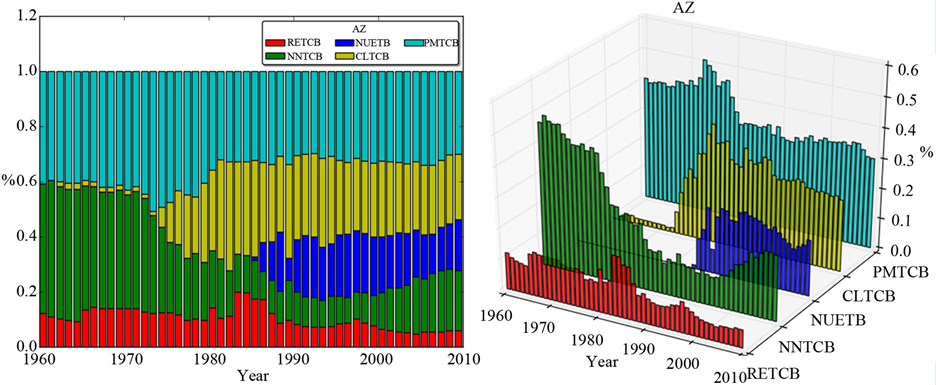Figure 1. Energy profile of AZ (data: TETCB of AZ)

California (CA)

New Mexico (NM)

Texas (TX)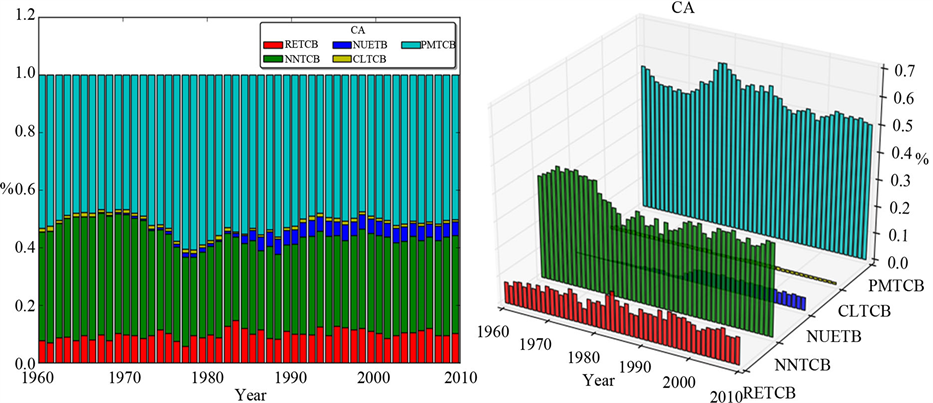Figure 2. Energy profile of CA (data: TETCB of CA)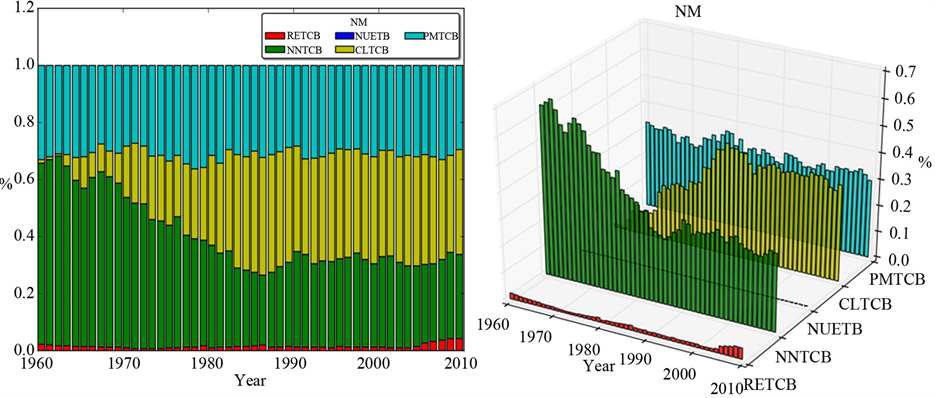Figure 3. Energy profile of NM (data: TETCB of NM)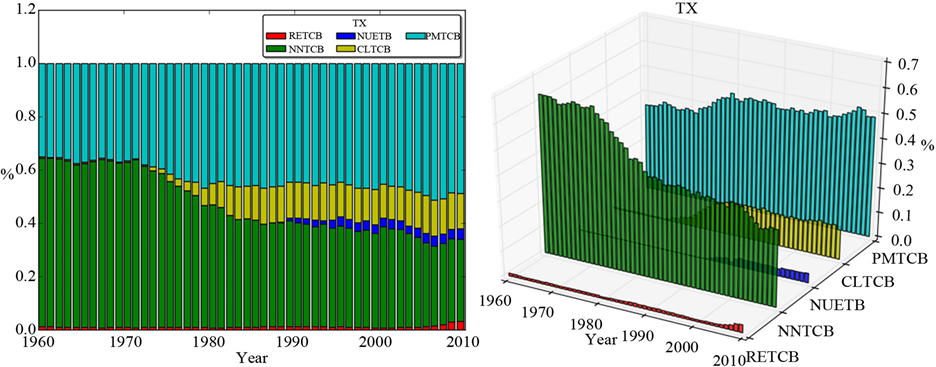Figure 4. Energy profile of TX (data: TETCB of TX)

4.2. 能量概况的历史演变和预测

4.2.1. ARIMA模型 

Ø 时间序列可视化

Ø 序列平稳(时间序列的预处理)

· 平稳的含义

· 将原序列转换为平稳序列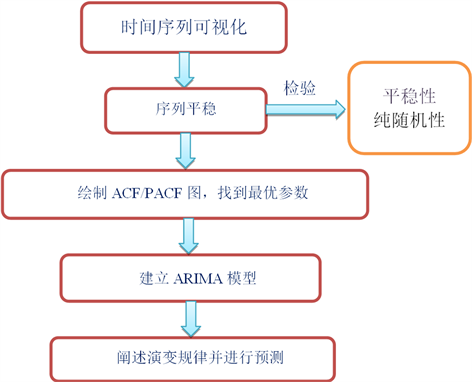Figure 5. Flow chart of ARIMA model

· 纯随机序列的处理

· 绘制ACF/PACF图，找到最优参数

4.2.2. 各州能源概况的演变规律

Ø 四州之间的相似性

Ø 四州之间的差异性

4.2.3. 各州能源概况的预测

Ø 预测结果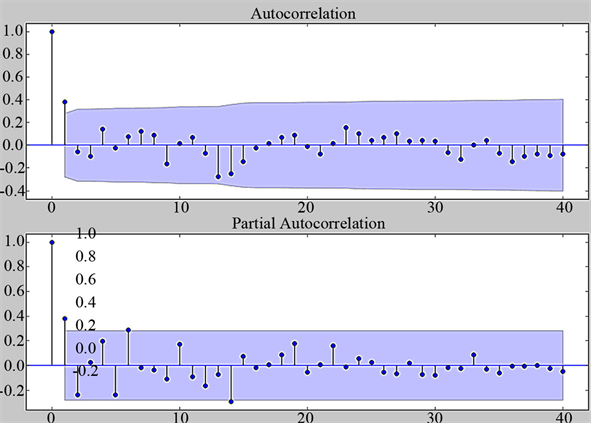Figure 6. The result of TETPBTX’s ACF&PACF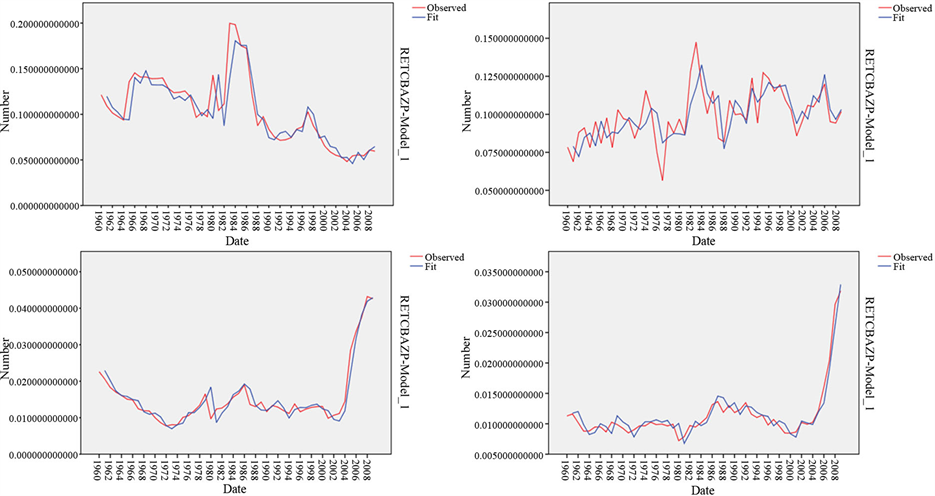*使用ARIMA模型得到的演变规律与原始数据相关系数R2分别为AZ：0.826，CA：0.454，NM：0.931，TX：0.928

Figure 7. The evolution of RETCP (percentage) in AZ, CA, NM & TX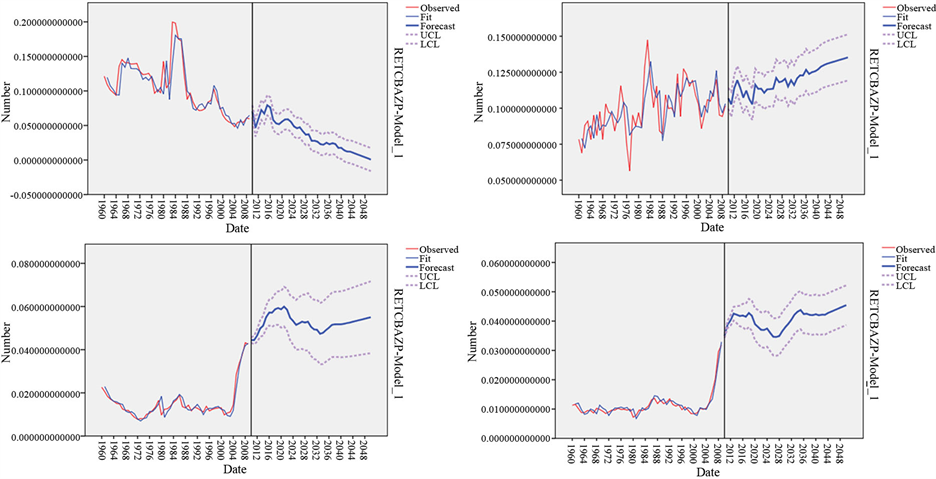Figure 8. The prediction of RETCP (percentage) in AZ, CA, NM & TX

4.3. 哪个州具有“最好”的清洁、可再生能源使用情况

$\begin{array}{l}T{R}_{1}\left(x\right)=\frac{\text{TETGR}\left(x\right)}{\sum \text{TETGR}\left(x\right)},x=\text{AZ},\text{CA},\text{NM},\text{TX}\\ T{B}_{1}\left(x\right)=\frac{\text{TETPB}\left(x\right)}{\sum \text{TETPB}\left(x\right)}\\ {C}_{2}\left(x\right)=\frac{\text{CLTCB}\left(x\right)}{\mathrm{max}\text{CLTCB}}\\ {P}_{2}\left(x\right)=\frac{\text{PMTCB}\left(x\right)}{\mathrm{max}\text{PMTCB}}\end{array}$Table 3. The result of the normalized model

$\begin{array}{l}T{R}_{2}\left(x\right)=\frac{T{R}_{1}\left(x\right)}{\mathrm{max}T{R}_{1}\left(x\right)}\\ T{B}_{2}\left(x\right)=\frac{T{B}_{1}\left(x\right)}{\mathrm{max}T{B}_{1}\left(x\right)}\\ {S}_{b}\left(x\right)=\mathrm{min}\left({C}_{2}\left(x\right)+{P}_{2}\left(x\right)+T{R}_{2}\left(x\right)+T{B}_{2}\left(x\right)\right)\end{array}$

5. 结语

 美国能源信息署[EB/OL]. https://www.eia.gov

 Cnblogs python时间序列分析[EB/OL]. https://www.cnblogs.com/foley/p/5582358.html

Top